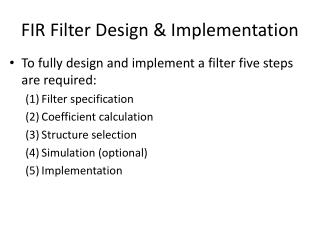DownloadDownload PresentationFIR Filter Design & Implementation

# FIR Filter Design & Implementation

Télécharger la présentation## FIR Filter Design & Implementation

- - - - - - - - - - - - - - - - - - - - - - - - - - - E N D - - - - - - - - - - - - - - - - - - - - - - - - - - -
##### Presentation Transcript

1. FIR Filter Design & Implementation • To fully design and implement a filter five steps are required: (1) Filter specification (2) Coefficient calculation (3) Structure selection (4) Simulation (optional) (5) Implementation

2. Software Tools for Designing Filter • MATLAB provides some advanced tools for filters design • Signal Processing Tool (SPTool) • Filter Design (FDATool) • There are also some free software packages to design filters • Our main problem is how to implement the filter given the coefficients • So we will design FIR filters using the relatively simple method of Windowing -- write Matlab scripts

3. Lowpass Filter Specification

4. Window Method : Step 1 • First stage of this method is to calculate the coefficients of the ideal filter. • This is calculated as follows:

5. Window Method : Step 2 • Second stage of this method is to select a window function based on the passband or attenuation specifications, then determine the filter length based on the required width of the transition band

6. Window Method : Step 2 • The normalized transition bandwidth as listed in the table is defined as • Example: If we choose the Hamming window, and specify a transition band from 6kHz to 7kHz, with sampling frequency at 40kHz, then the filter order is calculated as

7. Window Method : Step 3 • The third stage is to calculate the set of truncated or windowed impulse response coefficients, h[n]: for

8. Using Matlab's fir1() • Instead of doing the design start from the ideal reponse, we shall use Matlab's fir1() function • We will design a FIR filter with the following specification • sampling frequency = 8000 • passband edge frequency = 1200 • stopband edge frequency = 1800 • minimum stopband attenuation = 40 dB • By using the Hamming window, the stopband attenuation specification is met • By default, fir1() uses the Hamming window • For more information, please refer to the Matlab on-line help

9. Using Matlab's fir1() fsamp = 8000; fp = 1200; % passband fs = 1800; % stopband fc = (fp+fs)/2; % cutoff % default using Hamming window, compute order N = round(3.3*fsamp/(fs-fp)); str=sprintf('Filter order = %d', N); disp(str); wc = 2*fc/fsamp; % normalize to pi radian b=fir1(N,wc); freqz(b,1,512,fsamp); filtdesign.m

10. FIR Filter Structure - Direct Form

11. Linear Phase FIR • For linear phase FIR, such as those designed with the window method, the coefficients have to be symmetrical • We can then make use of the symmetry to reduce the multiplications

12. Example of Symmetrical FIR - Even Length

13. Example of Symmetrical FIR - Odd Length

14. FIR Filters Design with FDATool • The Filter Design and Analysis Tool (FDATool) is a graphical user interface (GUI) for designing, quantizing, and analyzing digital filters. • It includes a number of advanced filter design techniques and supports all the filter design methods in the Signal Processing Toolbox. • designing filters by setting filter specifications; • analyzing designed filters; • converting filters to different structures; and • quantizing and analyzing quantized filters. • Open the FDATool by typing "fdatool" at theMATLAB command window.